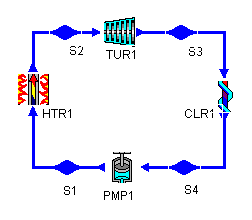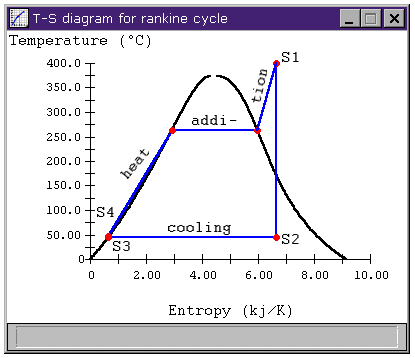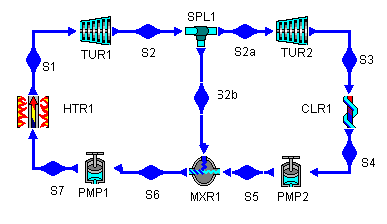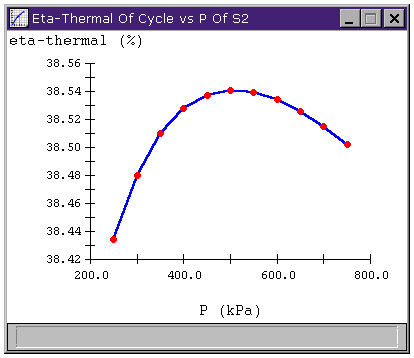Design Library

# Rankine Cycle with Regeneration

## Our Purpose

In thermodynamic power cycles, an improvement of even 0.5% in overall cycle efficiency is an important gain. One of the more common ways to improve the efficiency of a steam cycle is to use regeneration, a process where heat is taken from steam between turbine stages and used to heat water as it goes through pump stages. Using CyclePad, we will modify a Rankine cycle and examine the effects of regeneration on the cycle's thermal efficiency.

## The basic Rankine cycle

We will compare our regenerative cycle to a typical Rankine cycle. The Rankine cycle to which we will compare has the following parameters:

The operating limits are

• heater pressure of 5 MPa
• heater exit temperature of 400 C
• cooler pressure of 10 kPa
and its efficiencies are
• Carnot efficiency: 52.6%
• thermal efficiency: 36.2%

The regeneration cycle we examine will operate under the same limits. For reference, the Rankine cycle layout is shown below.Figure 1: a Rankine cycle

(For more details on constructing the Rankine cycle, see the
Rankine Cycle Setup page.)

## The Rankine Cycle with Rengeration

### Improving cycle efficiencies

Improving cycle efficiency almost always involves making a cycle more like a Carnot cycle operating between the same high and low temperature limits. The Carnot cycle is maximally efficient, in part, because it receives all of its heat addition at the same temperature, which is the highest temperature in the cycle. Similarly, it rejects all of its heat at the same low temperature. The T-s diagram below details the working of a Carnot cycle operating between the same temperature limits as our Rankine cycle.Figure 2: Carnot cycle T-s diagram

Most cycles don't have all of their heat addition or rejection at one temperature. So, when we look to improve a cycle's efficiency, we often consider the mean temperature of heat addition, Ta and the mean temperature of heat rejection, Tr. These reflect what the temperature would have been if the same amount of heat had been added (or rejected) all at one temperature. They allow us to treat improving cycle efficiencies as we would for a Carnot cycle: by raising Ta or lowering Tr. For reversable heat transfer, the average temperature of heat addition is

Ta = Qin / DS

and the average temperature of heat rejection is

Tr = Qout / DS

For more efficient cycles, we would like to add heat at a higher temperature and reject it at a lower temperature.Figure 3: Rankine cycle T-s diagram

Knowing this, let's look at Figure 3. In the Rankine cycle, the above equations tell us we are adding heat between states S4 and S1 at an average temperature of about 226.7 C. The heat rejection from S2 to S3 occurs at the cooler saturation temperature of 45.8 C. As a quick check, we can find the Rankine cycle's thermal efficiency by applying the relation for Carnot efficiency to the mean Rankine cycle temperatures:

h = (Ta - Tr) / Ta = (226.7 - 45.8) / (226.7+273.15) = 36.2%

Which is the same answer we get applying the usual h = Wnet / Qhi relation.

### How regeneration works

The idea behind regeneration is that we split the turbine into high-pressure and low-pressure stages and do the same for the pump. Then, we can divert some of the heat in the fluid as it leaves the high-pressure turbine and add it to the cool fluid leaving the low-pressure pump, thereby sending fluid with a higher temperature to the heater. We'll look at this in more detail in a minute, but now we know enough to construct the Rankine cycle with regeneration.Figure 4: a Rankine cycle with regeneration

## Choosing regeneration assumptions

With the design layout complete, we turn to adding the assumptions which allow CyclePad to solve the cycle. For this example, there are four places where we have to make new assumptions: the outlet of the high-pressure turbine (S2), the splitter (SPL1), the outlet of the low-pressure pump (PMP2), and the inlet of the high-pressure pump (PMP1). We will look at each of these in turn.

### The High-Pressure Turbine Outlet (S2)

What pressure do we choose for the extracted feedwater? We don't know yet, so we'll choose 200 kPa, which gives the two turbines pressure ratios of 25 and 20. This makes them roughly equal and keeps either one from having an astronomically high pressure ratio. (The original Rankine cycle had a turbine PR equal to 500!) Later, when we have the cycle solved and we can let CyclePad do sensitivity analyses, we will see if another pressure works better.

### The Splitter (SPL1)

The splitter is used to draw some of the working fluid from the high-pressure turbine stage and direct it towards the mixer. The assumption we make here is that the splitter is isoparametric. This means that the stuff exiting the splitter is the same as the stuff entering it.

#### Quick Note

The other assumption is that the splitter is not isoparametric. This simulates situations when we use a special splitter that allows us to separate the saturated mixture into two streams that each have different proportions of liquid and vapor. For instance, we could split 1 kg/sec, 60% quality stream into a pair of 0.4 kg/sec, 0% quality and a 0.6 kg/sec, 100% quality streams. This allows each stream to have different specific properties (v, h, and so on), though they still have the same temperature and pressure.

We might think that this would be advantageous to regeneration so that we could send only saturated vapor (which has higher enthalpy) to the low-pressure turbine and get more work out of it and send the low quality remainder down to heat the water entering the high-pressure pump. So why not do this? Let's try it. We can retract the isoparametric assumption and instead assume the stuff entering the low-pressure turbine is a saturated vapor. Then when our other assumptions for this cycle have been made, we will see that the efficiency has changed by a few hundredths of a percent and we see that such an approach does not improve cycle efficiency by much. Our high-pressure pump condition (S6 below) requires that the total enthalpy H (not h) entering the high-pressure pump is constant, so the lower quality of the steam entering the mixer requires a higher mass flow into the mixer, nearly negating the benefit of the higher h steam that enters the low-pressure turbine. That is, we could send lower h stuff to the pump, but we'd have to send more of it. Of course, any efficiency improvement is better than none, but it isn't always realistic to assume that we can use the special splitter than separates vapor from liquid, so we'll continue using the isoparametric splitter.

### The Low-pressure Pump Outlet (S5)

What pressure should the water at this state have? This water, which enters the pump at the cooler pressure, needs to be pumped up to the pressure of the water extracted from the high-pressure turbine. This is a matter of simple hydrostatics: if we make it lower, the higher pressure extract water will flow backward through the low-pressure pump and, if we make it higher, the low pressure pump water will flow backward through the splitter.

We could just set this pressure at 200 kPa, the pressure we set at the high-pressure turbine outlet. However, the better approach is to tell CyclePad to make the two pressures equal, using the "Equate T(S5) to another parameter" option. This way, when we need to experiment with different feedwater pressures, we don't need to make the change in two places. Another equivalent solution is to declare MXR1 to be isobaric.

### The High-Pressure Pump Inlet (S6)

Our whole purpose in adding heat to this water is to raise its temperature before it enters the heater and improve the cycle efficiency. The water exiting the low-pressure pump is only at 46 C and the water entering the heater in the original Rankine cycle was at about the same temperature (adding pressure to an incompressible fluid doesn't raise its temperature much). How high can we heat the water to improve this?

The only limit we really need to consider is the practical use of the pump. We make sure the water entering the pump in a simple Rankine cycle is a saturated fluid because pumps cannot handle vapor very well. We have the same consideration here. We want to heat this water up as much as we can, but not so much that some of it starts to vaporize again. We recall that this is the state of a saturated liquid. This is our assumption for the high-pressure pump inlet: it is saturated with quality = 0.

## Examining regeneration efficiencies

### The Effect on Cycle Efficiency

We now have made the changes needed to add a regeneration stage to our Rankine cycle. Here are the new efficiencies and the ones of the plain Rankine cycle for comparison. Also shown is the percent gain in power output of the modified cycle when supplied with the same heat as the unmodified Rankine cycle and the mean temperatures of heat addition for both cycles.

Cycle Efficiencies Unmodified Rankine
Cycle
Rankine Cycle
with Regeneration
Carnot efficiency 52.6% 52.6%
Thermal efficiency 36.2% 38.4%
% Increase in Power Generation 0% 6.1%
mean temperature of heat addition 226.7 C 251.5 C

### Varying Feedwater Pressure

Can we do even better? We made only one decision that was somewhat arbitrary in picking numbers, choosing the outlet pressure of the high-pressure turbine so that the two turbines would have similar (and low) pressure ratios. However, while that is a worthwhile choice considering the economics of turbine purchasing, it may not yield the optimal cycle efficiency.

We can examine the relationship between the thermal efficiency of our regenerative cycle and the feedwater pressure (the pressure at S2). The sensitivity analysis looks like thisFigure 5: Effect of varying feedwater pressure on
cycle efficiency of Rankine cycle with regeneration.

There appears to be an optimal pressure at about 500 kPa. It is important to pay attention to the scale of the graph, though, because, while there may be an increase in efficiency by changing the pressure, the efficiencies shown vary by roughly 0.1%, which may not justify the higher pressure ratio (about 50) needed in the low-pressure turbine to accommodate a feedwater pressure of 500 kPa.

Of course, the limit on the improvement made by the regeneration stage seems to be that we can only add so much heat to the fluid entering the high-pressure pump before the fluid begins to evaporate, damaging the pump. At higher pressures, the working fluid can contain more heat before it begins to boil away. So, we could repeat the process several times: add some heat until boiling almost occurs, then pump to a higher pressure, then add more heat, and so on.

For instance, by adding another regeneration stage to the design above, and hunting a little to find good feedwater pressures, we can improve the cycle's thermal efficiency to about 39.5%, which is a significant improvement from the 38.4% with one regeneration stage. A third regeneration stage brings the efficiency to just over 40%. How far can this go? For a Rankine cycle where the steam enters the boiler as a superheated gas, we can never achieve the efficiency of the Carnot cycle, even with an infinite number of regeneration stages. (If the steam entered the turbine as a saturated vapor, we could, in theory, achieve Carnot efficiency with infinite regeneration stages, but such cycles are impractical for other reasons besides the need for infinite stages.)

The real limit is economic, since having the extra extraction stages and pumps adds to the cost of the plant. At some point, the cost of the extra equipment outweighs the savings due to increased cycle efficiency. Practical plants seldom have more than five regeneration stages.

## Sources

Whalley, P.B. 1992. Basic Engineering Thermodynamics. Oxford University Press. ISBN: 0-19-856255-1

Haywood, R.W. 1980. Analysis of Engineering Cycles. Pergamon Press. ISBN: 0-08-025440-3

Contributed by: M. E. Brokowski
Initial Entry: 12/10/97
Last Edited: 12/15/97